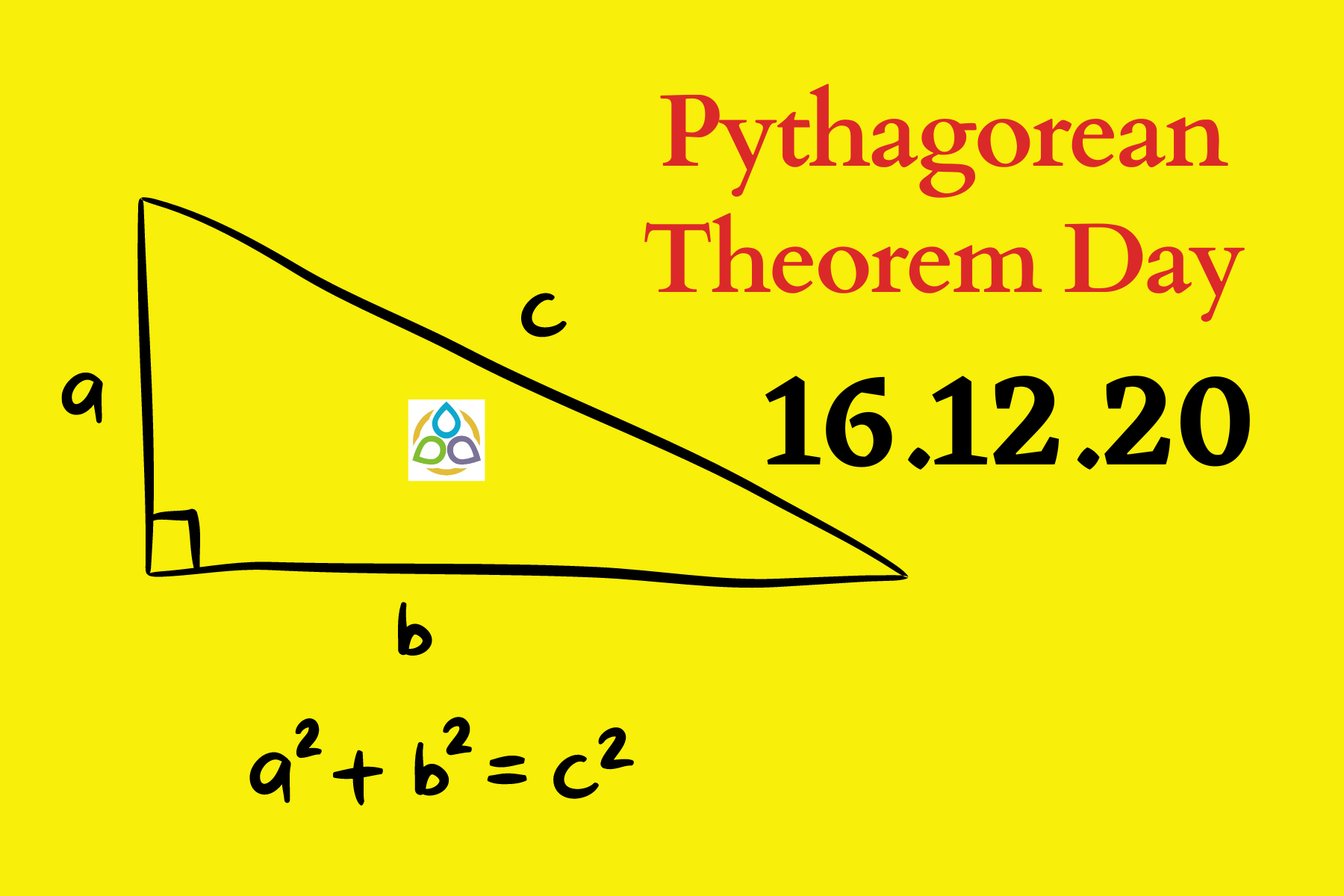# Pythagorean Theorem Day

Pythagoras was a philosopher and mathematician who lived around 500 BCE, and is known for his contributions to the field of mathematics, including the famous Pythagoras theorem which states that in a right triangle, the square of the length of the hypotenuse (the side opposite the right angle) is equal to the sum of the squares of the lengths of the other two sides. This can be written as the equation: c2 = a2 + b2, where c is the length of the hypotenuse and a, b are the lengths of the other two sides.

It is worth noting that many mathematical concepts and discoveries have been made throughout history on various days, and some of those days have been designated as mathematics days to commemorate and celebrate those achievements. For example, Pi Day on March 14th honours the mathematical constant Pi, and Fibonacci Day on November 23rd recognises the contributions of the mediaeval mathematician Fibonacci.

Pythagorean Theorem Day is celebrated when the sum of the squares of the first two digits in a date equals the square of the last digit in the date. The word Pythagorean refers to anything related to the ancient Greek mathematician Pythagoras or his followers, known as the Pythagoreans.

Today, December 16th, 2020, holds a specific significance within the field of mathematics. It is a day in which the sum of the squares of the first two digits (date and month) equals the square of the last two digits (of the year). Yes, today is a Pythagorean Theorem Day. We have

16² + 12² = 20² (256 + 144 = 400).

The last Pythagorean Theorem Day was on 15th August 2017, since

15² + 8² = 17² (225 + 64 = 289)

Pythagorean theorem days are of special interest because it doesn’t happen every year. In fact, we can’t find any such day in the next 4 years! The upcoming day will be 24.07.2025.